# Texas Go Math Grade 3 Lesson 2.3 Answer Key Fractions of a Whole

Refer to our Texas Go Math Grade 3 Answer Key Pdf to score good marks in the exams. Test yourself by practicing the problems from Texas Go Math Grade 3 Lesson 2.3 Answer Key Fractions of a Whole.

## Texas Go Math Grade 3 Lesson 2.3 Answer Key Fractions of a Whole

Essential Question
How does a fraction name part of a whole?

Unlock the Problem
The first pizzeria in America opened in New York in 1905. The pizza recipe came from Italy. Look at Italy’s flag. What fraction of the flag is not red?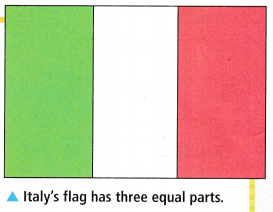Name equal parts of a whole.

A fraction can name more than 1 equal part of a whole.

The flag is divided into 3 equal parts, and 2 parts are not red.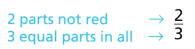Read: two thirds or two parts out of three equal parts
Write: $$\frac{2}{3}$$
So, ___ of the flag is not red.
So, $$\frac{2}{3}$$ of the flag is not red.

Math Idea
When all the parts are shaded, one whole shape is equal to all of its parts. It represents the whole number 1.
$$\frac{3}{3}$$ = 1

The numerator tells how many parts are being counted.
The denominator tells how many equal parts are in the whole or in the group.

You can count equal parts, such as sixths, to make a whole.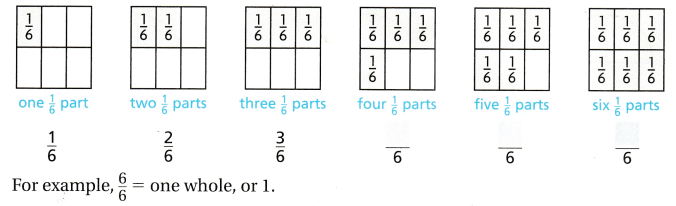Share and Show

Math Talk
Mathematical processes
Explain what the numerator denominator of a fraction tell you.
The numerator tells that the number of parts being counted. The denominator tells that the number of equal parts in the whole.

Question 1.
Shade two parts out of eight equal parts. Write a fraction in words and in numbers to name the shaded part.
Think: Each part is $$\frac{1}{8}$$.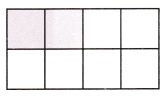Write: $$\frac{2}{8}$$

Explanation:
In the given question two parts out of eight equal parts are shaded. The fraction in words and in numbers to name the shaded part is Read: two eights and write: $$\frac{2}{8}$$.

Write the fraction that names each part. Write a fraction in words and in numbers to name the shaded part.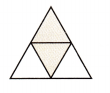Each part ___.
____ fourths
______
Each part is $$\frac{1}{4}$$
Two fourths
$$\frac{2}{4}$$

Explanation:
In the given question two parts out of four equal parts are shaded. The fraction in words and in numbers to name the shaded part is Read: two fourths and write: $$\frac{2}{4}$$.

Question 3.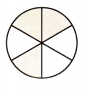Each part ___.
____ sixths
______
Each part is $$\frac{1}{6}$$
three sixths
$$\frac{3}{6}$$

Explanation:

In the given question three parts out of sixths equal parts are shaded. The fraction in words and in numbers to name the shaded part is Read: three sixths and write: $$\frac{3}{6}$$.

Question 4.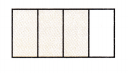Each part ___.
____ fourths
______
Each part $$\frac{1}{4}$$
three fourths
$$\frac{3}{4}$$

Explanation:
In the given question three parts out of four equal parts are shaded. The fraction in words and in numbers to name the shaded part is Read: three fourths and write: $$\frac{3}{4}$$.

Problem Solving

Shade the fraction circle to model the fraction. Then write the fraction in numbers.

Question 5.
three fourths$$\frac{3}{4}$$

Explanation:The

three out of three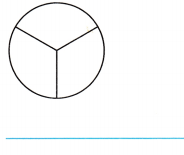$$\frac{3}{3}$$ or 1

Explanation:Question 7.
one out of two$$\frac{1}{2}$$

Explanation:Problem Solving

Shade the fraction circle to model the fraction. Then write the fraction in numbers.

Mrs. Ormond ordered pizza. Each pizza had 8 equal slices. Use the pictures for 8-11.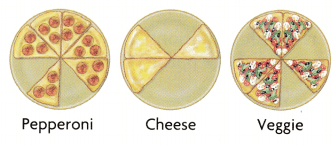Question 8.
What fraction of the pepperoni pizza was eaten?
$$\frac{2}{8}$$

Explanation:
Each pizza had 8 equal slices. Two slices of the pepperoni pizza was eaten. Therefore the fraction of the pepperoni pizza was eaten is $$\frac{2}{8}$$.

Question 9.
What fraction of the cheese pizza is left?
$$\frac{3}{8}$$

Explanation:
Each pizza had 8 equal slices. Three slices of the cheese pizza were eaten. Therefore the fraction of the cheese pizza was eaten is $$\frac{3}{8}$$.

Pose a Problem Use the picture of the veggie pizza to write a problem that includes a fraction. Solve your problem.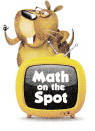Sadie ate $$\frac{3}{8}$$ of the veggie pizza. How many slices of veggie pizza did Sadie eat? 3 slices

Explanation:
By using the picture of the veggie pizza that includes a fraction is Sadie ate 3 8 of the veggie pizza.
And the question formed is How many slices of veggie pizza did Sadie eat? Therefore Sadie ate 3 slices.

Question 11.
Multi-Step Marcos ate $$\frac{1}{8}$$ of the cheese pizza, $$\frac{1}{8}$$ of the pepperoni pizza, and $$\frac{2}{8}$$ of the veggie pizza. How many slices of pizza did Marcos eat?
4 slices

Explanation:
Marcos ate the cheese pizza = $$\frac{1}{8}$$
pepperoni pizza = $$\frac{1}{8}$$
Veggie pizza = $$\frac{2}{8}$$
$$\frac{1}{8}$$+$$\frac{1}{8}$$+$$\frac{2}{8}$$ = $$\frac{4}{8}$$.
The number of slices of pizza Marcos ate is 4 slices.

Question 12.
What’s the Error? Kate says that $$\frac{2}{4}$$ of the rectangle is shaded. Describe her error. Write the correct fraction for the shaded part.Kate used the number of unshaded parts as the denominator. There are 6 equal parts and 2 are shaded, so $$\frac{2}{6}$$ is the correct fraction.

Explanation:In the given figure there are 6 equal parts and 2 parts are shaded. So the correct fraction for the shaded part is $$\frac{2}{6}$$ is the correct fraction.

Fill in the bubble for the correct answer choice.

Question 13.
Representations What fraction of this pizza garden contains peppers?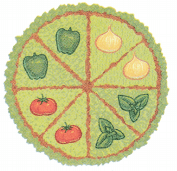(A) $$\frac{3}{8}$$
(B) $$\frac{2}{8}$$
(C) $$\frac{4}{8}$$
(D) $$\frac{2}{6}$$
$$\frac{2}{8}$$

Explanation:
The pizza contains 2 peppers out of 8. Therefore, $$\frac{2}{8}$$ fraction of the pizza contains pepper.

Use Diagrams Jane is making a memory quilt from some of her old favorite clothes that are too small. She will use T-shirts for the shaded squares in the pattern. Which names the part of the quilt that will be made of T-shirts?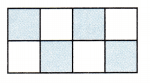(A) three fourths
(B) four sixths
(C) two eighths
(D) four eighths
four eighths

Explanation:

Question 15.
Multi-Step A pizza garden has six sections. Two sections are planted with tomatoes. Which fraction represents the part of the garden without tomatoes?
(A) $$\frac{2}{6}$$
(B) $$\frac{1}{6}$$
(C) $$\frac{4}{6}$$
(D) $$\frac{3}{6}$$
$$\frac{4}{6}$$

Texas Test Prep

Question 16.
What fraction names the shaded part of the shape?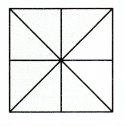(A) $$\frac{2}{8}$$
(B) $$\frac{8}{6}$$
(C) $$\frac{6}{8}$$
(D) $$\frac{2}{6}$$
$$\frac{6}{8}$$

Explanation:
By observing the shape we can find out 6 parts of the shape are shaded out of 8. So I have written $$\frac{6}{8}$$ fraction names the shaded part of the shape.

### Texas Go Math Grade 3 Lesson 2.3 Homework and Practice Answer Key

Shade the fraction circle to model the fraction.
Then write the fraction in numbers.

Question 1.
six out of eight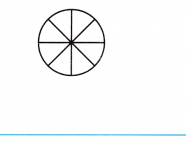$$\frac{6}{8}$$

Explanation:
The fraction in number is $$\frac{6}{8}$$.

Question 2.
five sixths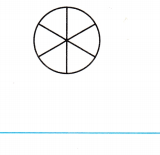$$\frac{5}{6}$$

Explanation:
The fraction in number is $$\frac{5}{6}$$.

Question 3.
one out of four$$\frac{1}{4}$$

Explanation:
The fraction in number is $$\frac{1}{4}$$.

two thirds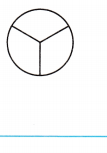$$\frac{2}{3}$$

Explanation:
The fraction in number is $$\frac{2}{3}$$.

Problem Solving

Question 5.
Shayna ordered a veggie pizza. The pizza had 8 slices. Shayna ate 3 slices. What fraction of the pizza is left?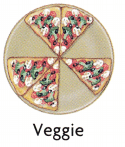$$\frac{5}{8}$$

Explanation:
Shayna ordered a pizza that had 8 slices. In those, she ate 3 slices. That means she ate $$\frac{3}{8}$$ of the pizza. The fraction that leftover pizza is 1-$$\frac{3}{8}$$= $$\frac{5}{8}$$

Lesson Check

Texas Test Prep

Question 6.
What fraction of the pizza garden contains onions?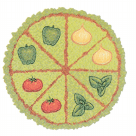(A) $$\frac{2}{6}$$
(B) $$\frac{2}{8}$$
(C) $$\frac{4}{8}$$
(D) $$\frac{3}{8}$$
$$\frac{2}{8}$$

Explanation:
The pizza contains 2 onions out of 8. Therefore, $$\frac{2}{8}$$ fraction of the pizza contains onions.

Question 7.
What fraction names the shaded part of the shape?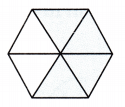(A) $$\frac{2}{6}$$
(B) $$\frac{4}{4}$$
(C) $$\frac{1}{6}$$
(D) $$\frac{4}{6}$$
$$\frac{4}{6}$$

Explanation:
The $$\frac{4}{6}$$ names the shaded part of the shape.

Question 8.
Gala plans to paint sections of a piece of paper with different colors. The diagram shows the colors she will use. What fraction of the piece of paper will be blue?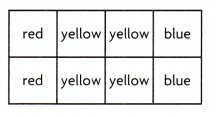(A) $$\frac{2}{8}$$
(B) $$\frac{2}{4}$$
(C) $$\frac{1}{8}$$
(D) $$\frac{4}{8}$$
$$\frac{3}{4}$$

Explanation:
Gala plans to paint a piece of paper with different colors. There are different colors she will use by using the diagram given. In the diagram, there are 2 blues and out of 8 colors. Hence the piece of paper that will be blue is 1- $$\frac{2}{8}$$ = $$\frac{3}{4}$$.

Multi-Step A flag is divided into four equal sections. One section is white. What fraction of the flag is not white?
(A) $$\frac{1}{4}$$
(B) $$\frac{2}{4}$$
(C) $$\frac{4}{4}$$
(D) $$\frac{3}{4}$$
$$\frac{3}{4}$$

Explanation:
The flag is divided into four equal sections = $$\frac{1}{4}$$
Here one section is white and the other is not white. So 1-$$\frac{1}{4}$$ = $$\frac{3}{4}$$

Question 10.
Multi-Step Two out of six equal sections of a flower garden contain daisies. The remaining sections contain different kinds of lilies. What fraction of the garden includes lilies?
(A) $$\frac{2}{6}$$
(B) $$\frac{4}{6}$$
(C) $$\frac{3}{6}$$
(D) $$\frac{1}{6}$$
$$\frac{4}{6}$$
The flower garden contain $$\frac{2}{6}$$ daisies
The fraction of the garden includes lilies is $$\frac{4}{6}$$.